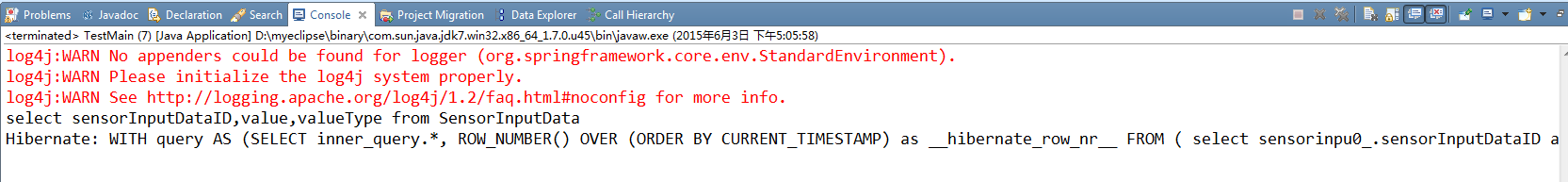`````` setInterval(function (){
\$.post(url,data,function()
{
do something
});
},2000);
``````

`````` String properties[]={"sensorInputDataID","value","valueType"};
List<Object[]> list=baceDao.getSpecifiedRanges(SensorInputData.class, properties,"201","1");
for(Object[] obj:list)
{
System.out.println("id="+obj.toString()+", value="+obj.toString()+",valueType="+obj.toString());
}
if(list==null)
{
System.out.println("null");
}
``````

`````` public List<Object[]> getSpecifiedRanges(Class<? extends Object> table,String[] properties,String startIndex,String rowCount)
{
int propertysLength= properties.length;
String hql="select ";
for(int i=0;i<propertysLength-1;i++)
{
hql+=properties[i]+",";
}
hql+=properties[propertysLength-1]+" from "+table.getSimpleName();

System.out.println(hql);
List<Object[]> result=sessionFactory.getCurrentSession().createQuery(hql).setFirstResult(Integer.parseInt(startIndex)).setMaxResults(Integer.parseInt(rowCount)).list();
return result;
}
``````6个回答

1、setInterval前select count(*) 一次获取总数，然后记录数量，在循环N次取数
2、每次取一条，如果没有记录了后台返回null，前端就知道没数据了，只是得多取一次才知道。4 年多之前 回复